﻿ 后掠式叶轮污泥回流泵的优化设计与数值模拟 The Optimal Design and Numerical Simulation of the Swept-Back Blade Sludge Return Pump

International Journal of Fluid Dynamics
Vol. 06  No. 04 ( 2018 ), Article ID: 27745 , 8 pages
10.12677/IJFD.2018.64011

The Optimal Design and Numerical Simulation of the Swept-Back Blade Sludge Return Pump

Bin Chen1, Yang Ma1, Zhenxing Sun1, Jialin Yang2, Qiujing Jin3

1Institute of Chemical Machinery of School of Mechanical Engineer, Hefei University of Technology, Hefei Anhui

2Lanshen Group Co. Ltd., Nanjing Jiangsu

3Nanjing Hegong Fluid Technology Co. Ltd., Nanjing Jiangsu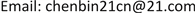Received: Nov. 2nd, 2018; accepted: Nov. 20th, 2018; published: Nov. 27th, 2018ABSTRACT

In view of the return sludge pump’s winding problem in the sewage, this paper designs three sweepback angles of blades: 20˚, 40˚, 60˚; Aiming at the problem of low efficiency of the unit, two types of guide vanes: segmental type and twisted type, are redesigned. This paper compared and analyzed the performance of the pump before and after optimization separately from the external characteristics and the internal flow field distribution based on RNG k-ε turbulent model and SIMPLE algorithm. Numerical simulation results show that with the increase of the blade swept angle, the lift and shaft power falls and as the Angle increases, so does the drop; the efficiency curve of 20˚ back swept blade is smooth and higher than that of the prototype pump. The modification of the distorted guide blade has greatly improved the lift and efficiency of the reflux pump.

Keywords:Sludge Reflux Pump, Back Swept Blade, Guide Vane Design

1合肥工业大学机械工程学院化工机械研究所，安徽 合肥

2蓝深集团股份有限公司，江苏 南京

3南京合工流体科技有限公司，江苏 南京1. 引言

2. 物理模型与数值计算

2.1. 物理模型及网格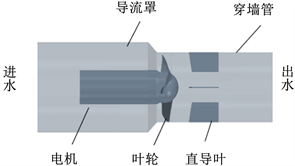Figure 1. Three-dimensional model of sludge return pump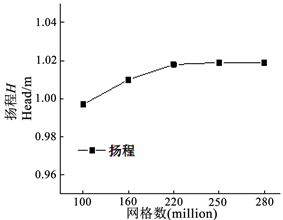Figure 2. Grid independence check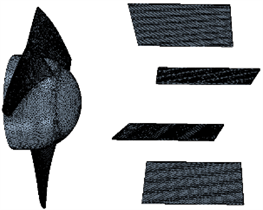Figure 3. Meshes of hydraulic components

2.2. 控制方程

$\frac{\partial \left(\rho \phi \right)}{\partial t}+\text{div}\rho u\phi =div\Gamma grad\phi +S$

$\frac{\partial \left(\rho \phi \right)}{\partial t}+\frac{\partial \rho u\phi }{\partial x}+\frac{\partial \rho v\phi }{\partial y}+\frac{\partial \rho w\phi }{\partial z}=\frac{\partial }{\partial x}\left(\Gamma \frac{\partial \phi }{\partial x}\right)+\frac{\partial }{\partial y}\left(\Gamma \frac{\partial \phi }{\partial y}\right)+\frac{\partial }{\partial z}\left(\Gamma \frac{\partial \phi }{\partial z}\right)+S$

2.3. 湍流模型和边界条件

3. 数值计算及结果分析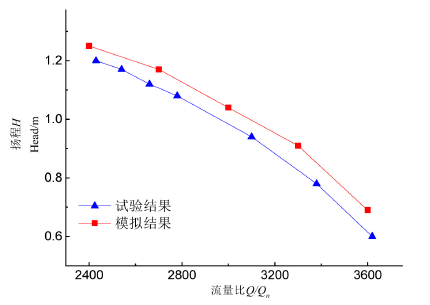Figure 4. Comparison of simulation and experimental values of the prototype

4. 后掠式污泥回流泵叶片优化设计

4.1. 后掠设计方法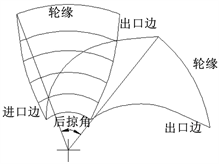Figure 5. Back swept angle of impeller

4.2. 数值模拟结果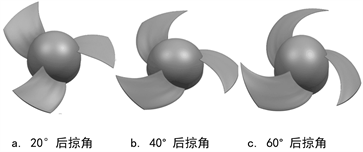Figure 6. Three-dimensional model of impellers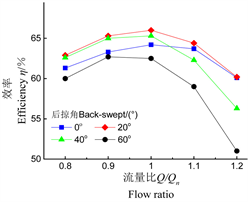Figure 7. Performance curves of different back-swept impellers

5. 导叶改型对污泥回流泵性能影响

5.1. 直导叶及改型导叶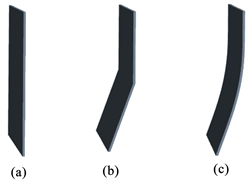Figure 8. Three-dimensional model of guide vane, (a) Straight, (b) sectional, (c) distorted

5.2. 三种导叶流线图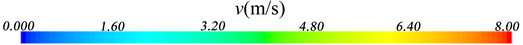Figure 9. Streamline graph of three guide vanes

5.3. 导叶改型对外特性影响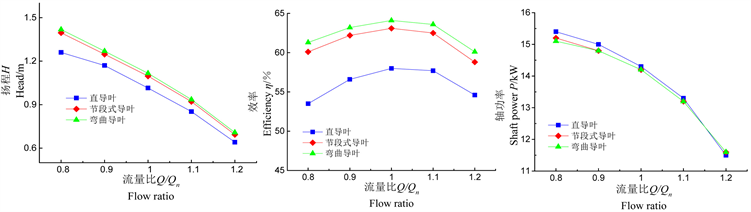Figure 10. Comparison of characteristic curves of different guide vane schemes

6. 结论

1) 叶片的后掠降低了污泥回流泵的扬程与轴功率，并且随着叶片后掠角度的增大，扬程与轴功率下降幅度更大。

2) 随着叶片后掠角的变化，污泥回流泵效率曲线变化并不规律；后掠角为20˚的污泥回流泵效率在各个流量下均高于原型泵。

3) 扭曲导叶方案提高了污泥回流泵的扬程和效率，并且能较好的改善导叶内液流的流动情况，提高污泥回流泵整体性能。

The Optimal Design and Numerical Simulation of the Swept-Back Blade Sludge Return Pump[J]. 流体动力学, 2018, 06(04): 85-92. https://doi.org/10.12677/IJFD.2018.64011

1. 1. 谷文涛, 张纯德, 戴文权. 三叶后掠式搅拌器的设计开发及其应用[J]. 聚氯乙烯, 1998(2): 1-6.

2. 2. 夏永忠, 高峰, 吴进, 等. 潜水搅拌器(推流器)结构优化[J]. 泰州职业技术学院学报, 2011, 11(5): 71-73.

3. 3. 王小云. 后掠式双叶片污水泵优化设计与试验研究[J]. 科技资讯, 2015, 13(12): 110.

4. 4. 郭龙凯, 刘艳明, 崔庆, 等. 叶片前缘不同后掠角对离心压气机气动性能的影响[J]. 热能动力工程, 2016, 31(4): 45-50.

5. 5. 王峰. 低风速风力机弯掠叶片优化及CFD分析[D]: [硕士学位论文]. 扬州: 扬州大学, 2017: 49-60.

6. 6. 郎涛, 施卫东, 邢津, 等. 相同外特性的后掠式轴流泵固液两相分布与缠绕试验[J]. 农业工程学报, 2015(23): 42-50.

7. 7. Larwood, S.M. and Zuteck, M.D. (2006) Swept Wind Turbine Blade Aeroelastic Modeling for Loads and Dynamic Behavior. American Wind Energy Association Windpower, Pittsburgh.

8. 8. Chattot, J. (2009) Effects of Blade Tip Mod-ifications on Wind Turbine Performance Using Vortex Model. Computers & Fluids, 38, 1405-1410. https://doi.org/10.1016/j.compfluid.2008.01.022

9. 9. 王福军. 计算流体动力学分析——CFD软件原理与应用[M]. 北京: 清华大学出社, 2004.